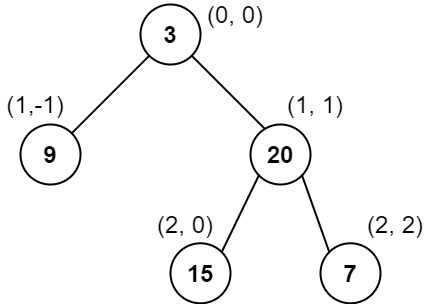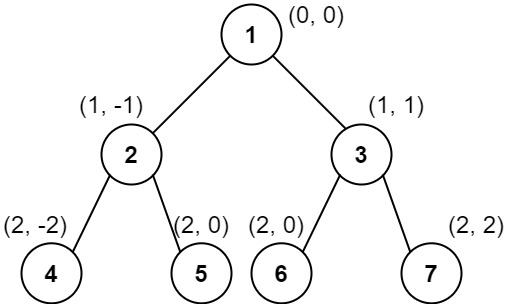## Algorithm

Problem Name: 987. Vertical Order Traversal of a Binary Tree

Given the `root` of a binary tree, calculate the vertical order traversal of the binary tree.

For each node at position `(row, col)`, its left and right children will be at positions `(row + 1, col - 1)` and `(row + 1, col + 1)` respectively. The root of the tree is at `(0, 0)`.

The vertical order traversal of a binary tree is a list of top-to-bottom orderings for each column index starting from the leftmost column and ending on the rightmost column. There may be multiple nodes in the same row and same column. In such a case, sort these nodes by their values.

Return the vertical order traversal of the binary tree.

Example 1:```Input: root = [3,9,20,null,null,15,7]
Output: [,[3,15],,]
Explanation:
Column -1: Only node 9 is in this column.
Column 0: Nodes 3 and 15 are in this column in that order from top to bottom.
Column 1: Only node 20 is in this column.
Column 2: Only node 7 is in this column.```

Example 2:```Input: root = [1,2,3,4,5,6,7]
Output: [,,[1,5,6],,]
Explanation:
Column -2: Only node 4 is in this column.
Column -1: Only node 2 is in this column.
Column 0: Nodes 1, 5, and 6 are in this column.
1 is at the top, so it comes first.
5 and 6 are at the same position (2, 0), so we order them by their value, 5 before 6.
Column 1: Only node 3 is in this column.
Column 2: Only node 7 is in this column.
```

Example 3:```Input: root = [1,2,3,4,6,5,7]
Output: [,,[1,5,6],,]
Explanation:
This case is the exact same as example 2, but with nodes 5 and 6 swapped.
Note that the solution remains the same since 5 and 6 are in the same location and should be ordered by their values.
```

Constraints:

• The number of nodes in the tree is in the range `[1, 1000]`.
• `0 <= Node.val <= 1000`

## Code Examples

### #1 Code Example with C Programming

```Code - C Programming```

``````
typedef struct {
int val;
int x;
int y;
} elem_t;
void traversal(elem_t **p, int *sz, int *n, struct TreeNode *node, int x, int y) {
if (!node) return;
if (*sz == *n) {
*sz *= 2;
*p = realloc(*p, (*sz) * sizeof(elem_t));
//assert(*p);
}
(*p)[*n].val = node->val;
(*p)[*n].x   = x;
(*p)[*n].y   = y;
(*n) ++;
traversal(p, sz, n, node->left,  x - 1, y + 1);
traversal(p, sz, n, node->right, x + 1, y + 1);
}
int cmp(const void *a, const void *b) {
elem_t *ea = a, *eb = b;
int k = ea->x - eb->x;
if (k == 0) k = ea->y - eb->y;
if (k == 0) k = ea->val - eb->val;
return k;
}
int** verticalTraversal(struct TreeNode* root, int** columnSizes, int* returnSize) {
elem_t *p;
int sz, n;
int **ret, *buff, buff_sz, i, j, k;

if (!root) return NULL;

sz = 100;
p = malloc(sz * sizeof(elem_t));
//assert(p);
n = 0;

// put all nodes into the array
traversal(&p, &sz, &n, root, 0, 0);

// sort all nodes according to x and y
qsort(p, n, sizeof(elem_t), cmp);

// make the array for return
k = p[n - 1].x - p.x + 1;
ret = malloc(k * sizeof(int *));
(*columnSizes) = calloc(k, sizeof(int));
//assert(ret && *columnSizes);

*returnSize = k;

j = k = 0;
for (i = 0; i < n; i ++) {
//printf("%d, ", p[i].val);
if (j == 0 || p[i].x != p[i - 1].x) {
j = 0;
buff_sz = 10;
buff = malloc(buff_sz * sizeof(int));
//assert(buff);
ret[k ++] = buff;
}
if (buff_sz == j) {
buff_sz *= 2;
buff = realloc(buff, buff_sz * sizeof(int));
//assert(buff);
ret[k - 1] = buff;
}
buff[j ++] = p[i].val;
(*columnSizes)[k - 1] = j;
}

free(p);

return ret;
}
``````
Copy The Code &

Input

cmd
root = [3,9,20,null,null,15,7]

Output

cmd
[,[3,15],,]

### #2 Code Example with Javascript Programming

```Code - Javascript Programming```

``````
const verticalTraversal = function(root) {
const arr = []
helper(root, 0, 0, arr)
arr.sort((a, b) => a - b || b - a || a - b)
const res = new Map()

for(let [x, y, val] of arr) {
if(!res.has(x)) res.set(x, [])
res.get(x).push(val)
}
return [...res.values()]
};

function helper(node, x, y, arr) {
if(node) {
helper(node.left, x - 1, y - 1, arr)
arr.push([x, y, node.val])
helper(node.right, x  + 1, y - 1, arr)
}
}
``````
Copy The Code &

Input

cmd
root = [3,9,20,null,null,15,7]

Output

cmd
[,[3,15],,]

### #3 Code Example with Python Programming

```Code - Python Programming```

``````
class Solution:
def verticalTraversal(self, root: TreeNode) -> List[List[int]]:
self.arr = []
def dfs(node, x, y):
if node:
self.arr.append((x, y, node.val))
dfs(node.left, x - 1, y + 1)
dfs(node.right, x + 1, y + 1)
dfs(root, 0, 0)
return [list(map(lambda x: x[-1], g)) for k, g in itertools.groupby(sorted(self.arr), key = lambda x: x)]
``````
Copy The Code &

Input

cmd
root = [1,2,3,4,5,6,7]

Output

cmd
[,,[1,5,6],,]

### #4 Code Example with C# Programming

```Code - C# Programming```

``````
using System.Collections.Generic;
using System.Linq;

namespace LeetCode
{
public class _0987_VerticalOrderTraversalOfABinaryTree
{
public IList> VerticalTraversal(TreeNode root)
{
var results = new List>();
if (root == null) return results;

var columnTable = new Dictionary>();
var queue = new Queue<(int col, TreeNode node)>();
queue.Enqueue((0, root));

while (queue.Count > 0)
{
var size = queue.Count;
var levelColumnTable = new Dictionary>();
for (int i = 0; i < size; i++)
{
(int col, TreeNode node) = queue.Dequeue();

if (!levelColumnTable.ContainsKey(col))
levelColumnTable[col] = new List();

if (node.left != null)
queue.Enqueue((col - 1, node.left));
if (node.right != null)
queue.Enqueue((col + 1, node.right));
}

foreach (var pair in levelColumnTable)
{
if (!columnTable.ContainsKey(pair.Key))
columnTable[pair.Key] = new List();

}
}

var min = columnTable.Keys.Min();
var max = columnTable.Keys.Max();
for (int i = min; i <= max; i++)

return results;
}
}
}
``````
Copy The Code &

Input

cmd
root = [1,2,3,4,5,6,7]

Output

cmd
[,,[1,5,6],,]